## Example Questions

### Example Question #1 : Applying Triangle Similarity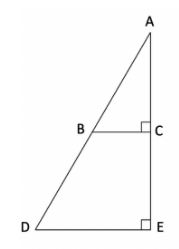In the above figure, line segment AB measures 10, line segment AC measures 8, line segment BD measures 10, and line segment DE measures 12. What is the perimeter of trapezoid BCDE?

36

28

32

24

36

Explanation:

As you unpack the given information, a few things should stand out:

1. Triangles ABC and ADE are similar. They each have a right angle and they each share the angle at point A, meaning that their lower-left-hand angles (at points B and D) will be the same also since all angles in a triangle must sum to 180.

2. Side BC has to measure 6, as you’re given one side (AC = 8) and the hypotenuse (AB = 10) of a right triangle. You can use Pythagorean Theorem to solve, or you can recognize the 3-4-5 side ratio (which here amounts to a 6-8-10 triangle).

3. This then allows you to use triangle similarity to determine the side lengths of the large triangle. Since the hypotenuse is 20 (segments AB and BD, each 10, combine to form a side of 20) and you know it’s a 3-4-5 just like the smaller triangle, you can fill in side DE as 12 (twice the length of BC) and segment CE as 8.

So you now know the dimensions of the parallelogram: BD is 10, BC is 6, CE is 8, and DE is 12. The sum of those four sides is 36.

### Example Question #1 : Triangles, Lines, & Angles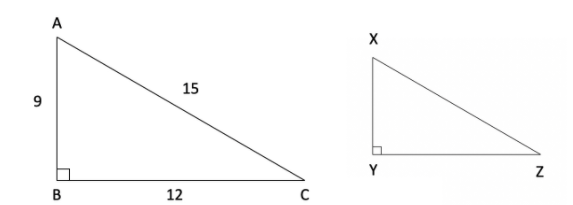In the figure above, triangle ABC is similar to triangle XYZ.  If side XZ measures 10, what is the area of triangle XYZ?

24

40

48

32

24

Explanation:

Because the triangles are similar, you can tell that if the hypotenuse of the larger triangle is 15 and the hypotenuse of the smaller triangle is 10, then the sides have a ratio of 3:2 between the triangles.  This allows you to fill in the sides of XYZ: side XY is 6 (which is 2/3 of its counterpart side AB which is 9) and since YZ is 8 (which is 2/3 of its counterpart side, BC, which is 12).

Since the area of a triangle is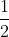Base * Height, if you know that you have a base of 8 and a height of 6, that means that the area is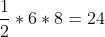.

### Example Question #2 : Applying Triangle Similarity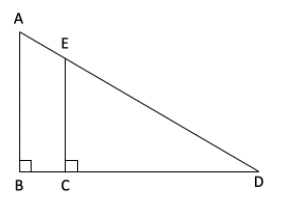In the triangle above, line segment BC measures 2 and line segment CD measures 8. If the area of triangle ABD is 25, then what is the length of line segment EC?

4

3

5

6

4

Explanation:

One important concept to recognize in this problem is that the triangles ABD and ECD are similar. Each has a right angle and they share the same angle at point D, meaning that their third angles (BAD and CED, the angles at the upper left of each triangle) must also have the same measure.

With that knowledge, you can use the given side lengths to establish a ratio between the side lengths of the triangles. If BC is 2 and CD is 8, that means that the bottom side of the triangles are 10 for the large triangle and 8 for the smaller one, or a 5:4 ratio.

You’re then told the area of the larger triangle. Knowing that the area is 25 and that area =Base x Height, you can plug in 10 as the base and determine that the height, side AB, must be 5.

Since you know that the smaller triangle’s height will be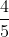the length of 5, you can then conclude that side EC measures 4, and that is your right answer.

### Example Question #4 : Applying Triangle Similarity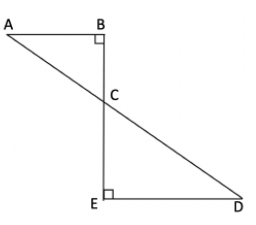Lines AD and BE intersect at point C as pictured. Which of the following ratios is equal to the ratio of the length of line segment AB to the length of line segment AC?

Side length CD to side length CE

Side length ED to side length CD

Side length CE to side length ED

Side length ED to side length CE

Side length ED to side length CD

Explanation:

In beginning this problem, it is important to note that the two triangles pictured, ABC and CED, are similar. They each have a right angle and they share the vertical angle at point C, meaning that the angles at A and D must also be congruent and therefore the triangles are similar.

This means that their side lengths will be proportional, allowing you to answer this question. You’re asked to match the ratio of AB to AC, which are the side across from angle C and the hypotenuse, respectively.  In triangle CED, those map to side ED and side CD, so the ratio you want is ED:CD.

### Example Question #4 : Triangles, Lines, & Angles

Triangle ABC is similar to triangle DEF. If the perimeter of triangle ABC is twice the length of the perimeter of triangle DEF, what is the ratio of the area of triangle ABC to the area of triangle DEF?

1:2

1:4

3:1

4:1

4:1

Explanation:

If the perimeter of triangle ABC is twice as long as the perimeter of triangle DEF, and you know that the triangles are similar, that then means that each side length of ABC is twice as long as its corresponding side in triangle DEF. That also means that the heights have the same 2:1 ratio: the height of ABC is twice the length of the height of DEF.

Since the formula for area of a triangle isBase x Height, you can express the area of triangle DEF asbh and the area of ABC as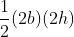.  You’ll then see that the areas of ABC to DEF are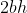andbh, for a ratio of 4:1.

NOTE: It can seem surprising that the ratio isn’t 2:1 if each length of one triangle is twice its corresponding length in the other. But keep in mind that for an area you multiply two lengths together, and go from a unit like “inches” to a unit like “square inches.”  Because each length is multiplied by 2, the effect is exacerbated.

### Example Question #1 : Applying Triangle Similarity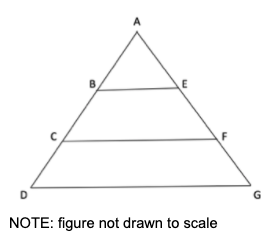In the figure above, lines DG, CF, and BE are parallel.  If line segment AB = 6, line segment AE = 9, line segment EF = 10, and line segment FG = 11, what is the length of line AD?

23

22

21

20

20

Explanation:

A key to solving this problem comes in recognizing that you’re dealing with similar triangles. Because lines BE, CF, and DG are all parallel, that means that the top triangle ABE is similar to two larger triangles, ACF and ADG. You know that because they all share the same angle A, and then if the horizontal lines are all parallel then the bottom two angles of each triangle will be congruent as well. You’ve established similarity through Angle-Angle-Angle.

This means that the side ratios will be the same for each triangle.  And for the top triangle, ABE, you know that the ratio of the left side (AB) to right side (AE) is 6 to 9, or a ratio of 2 to 3.  Note then that the remainder of the given information provides you the length of the entire right-hand side, line AG, of larger triangle ADG.  If AE is 9, EF is 10, and FG is 11, then side AG is 30.  And since you know that the left-hand side has a 2:3 ratio to the right, then line segment AD must be 20.

### Example Question #1 : Applying Triangle Similarity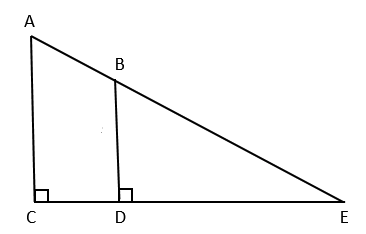In the figure above, line segment AC is parallel to line segment BD. If line segment AC = 15, line segment BD = 10, and line segment CE = 30, what is the length of line segment CD?

25

15

20

10

10

Explanation:

This problem tests the concept of similar triangles. First, you should recognize that triangle ACE and triangle BDE are similar. You know this because they each have the same angle measures: they share the angle created at point E and they each have a 90-degree angle, so angle CAE must match angle DBE (the top left angle in each triangle.

Because these triangles are similar, their dimensions will be proportional. Since sides, AC and BD - which are proportional sides since they are both across from the same angle, E - share a 3:2 ratio you know that each side of the smaller triangle (BDE) will be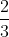as long as its counterpart in the larger triangle (ACE). Consequently, if the bottom side CE in the larger triangle measures 30, then the proportional side for the smaller triangle (side DE) will beas long, measuring 20.

Since the question asks for the length of CD, you can take side CE (30) and subtract DE (20) to get the correct answer, 10.

### Example Question #8 : Applying Triangle Similarity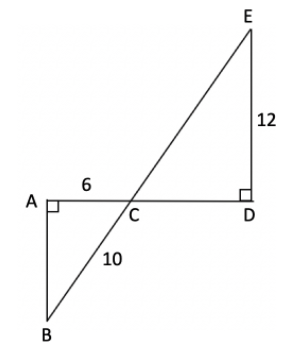In the figure above, line segments AD and BE intersect at point C. What is the length of line segment BE?

25

28

18

20

25

Explanation:

This problem hinges on your ability to recognize two important themes: one, that triangle ABC is a special right triangle, a 6-8-10 side ratio, allowing you to plug in 8 for side AB. And secondly, triangles ABC and CDE are similar triangles. You know this because each triangle is marked as a right triangle and angles ACB and ECD are vertical angles, meaning that they’re congruent. Since all angles in a triangle must sum to 180, if two angles are the same then the third has to be, too, so you’ve got similar triangles here.

With that knowledge, you know that triangle ECD follows a 3-4-5 ratio (the simplified version of 6-8-10), so if the side opposite angle C in ABC is 8 and in CDE is 12, then you know you have a 9-12-15 triangle. With the knowledge that side CE measures 15, you can add that to side BC which is 10, and you have the answer of 25.

### Example Question #6 : Triangles, Lines, & Angles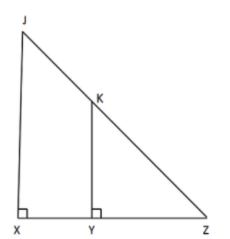In the diagram above, line JX is parallel to line KY. If JX measures 16, KY measures 8, and the area of triangle JXZ is 80, what is the length of line segment XY?

10

5

6

8

5

Explanation:

An important point of recognition on this problem is that triangles JXZ and KYZ are similar. Each has a right angle and each shares the angle at point Z, so the third angles (XJZ and YKZ, each in the upper left corner of its triangle) must be the same, too.

Given that, if you know that JX measures 16 and KY measures 8, you know that each side of the larger triangle measures twice the length of its counterpart in the smaller triangle.

You also have enough information to solve for side XZ, since you’re given the area of triangle JXZ and a line, JX, that could serve as its height (remember, to use thebase x height equation for area of a triangle, you need base and height to be perpendicular; lines JX and XZ are perpendicular). Since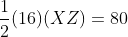, you can see that XZ must measure 10. And since XZ will be twice the length of YZ by the similarity ratio, YZ = 5, meaning that XY must also be 5.

### Example Question #1 : Applying Triangle Similarity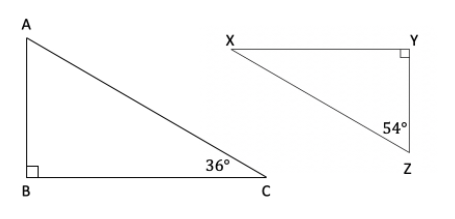For the pictured triangles ABC and XYZ, which of the following is equal to the ratio?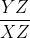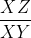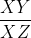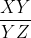Explanation:

The first important thing to note on this problem is that for each triangle, you’re given two angles: a right angle, and one other angle. Because all angles in a triangle must sum to 180 degrees, this means that you can solve for the missing angles.

In ABC, you have angles 36 and 90, meaning that to sum to 180 the missing angle ACB must be 54. And in XYZ, you have angles 90 and 54, meaning that the missing angle XZY must be 36.

Next, you can note that both triangles have the same angles: 36, 54, and 90.  This means that the triangles are similar, which also means that their side ratios will be the same. You just need to make sure that you’re matching up sides based on the angles that they’re across from.

You’re given the ratio of AC to BC, which in triangle ABC is the ratio of the side opposite the right angle (AC) to the side opposite the 54-degree angle (BC). In triangle XYZ, those sides are XZ and XY, so the ratio you’re looking for is.

### All SAT Mathematics Resources